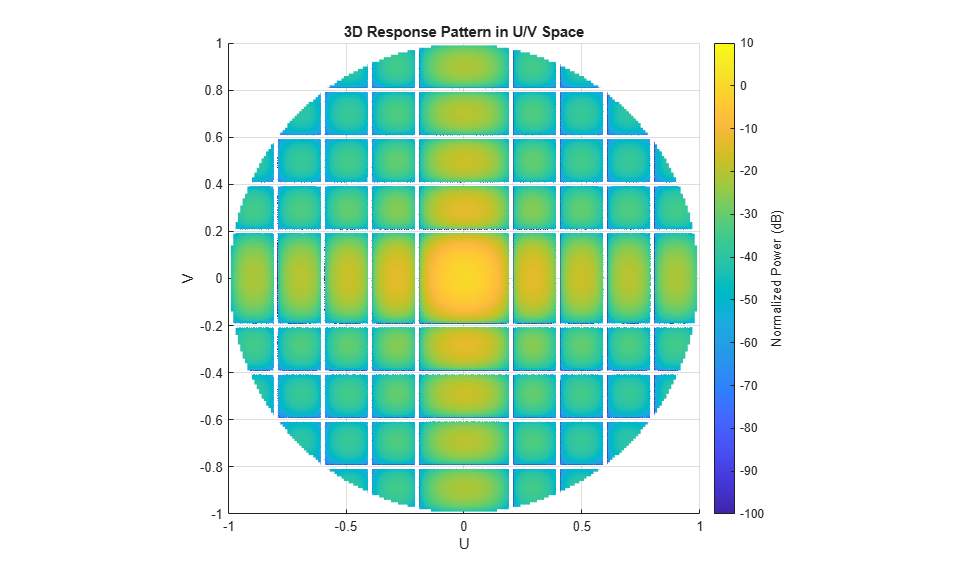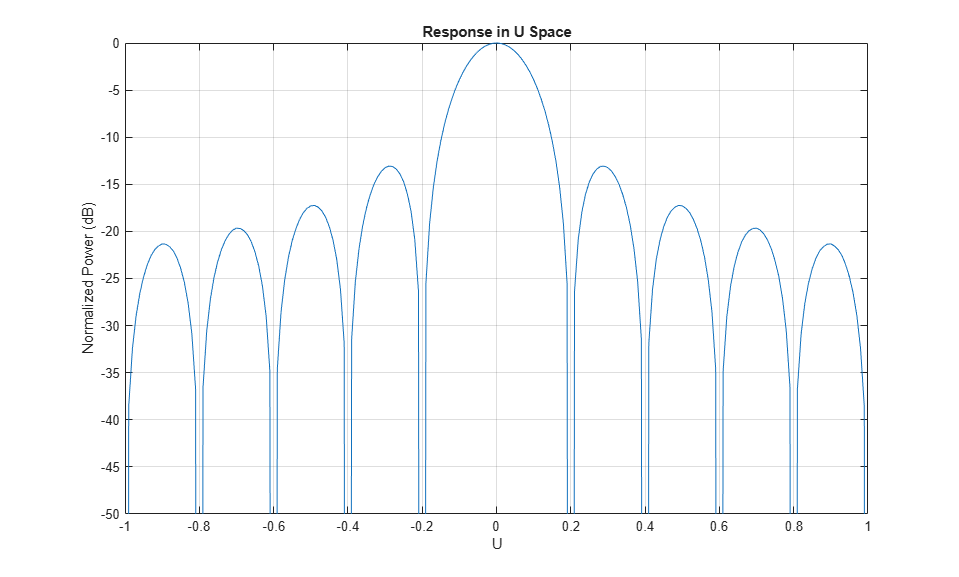Documentation

This is machine translation

Mouseover text to see original. Click the button below to return to the English version of the page.

Antenna Array Analysis with Custom Radiation Pattern

This example shows how to form an antenna array with a custom antenna radiation pattern and then analyze the array's response pattern. Such a pattern can be either from measurement or from simulation.

Depending on the application, practical phased antenna arrays sometimes use specially designed antenna elements whose radiation pattern cannot be represented by a closed-form equation. In addition, even when the element pattern is well understood, as is the case with a dipole antenna, the mutual coupling among the elements can significantly change the individual element pattern when the element is put into an array. This makes the closed-form pattern less accurate. Thus, for high fidelity pattern analysis, one often needs to use a custom radiation pattern obtained from measurements or simulations.

A frequently-used full-wave modeling tool for simulating antenna radiation patterns is HFSS™. In this tool, individual elements are modeled as if they were part of an infinite array. The simulated radiation pattern is represented as an M-by-3 matrix whose first column represents angle, whose second column represents angle, and whose third column represents the radiation pattern in dB. The coordinate system, or more specifically, the definition ofandused in HFSS is shown in Figure 1a. In this convention, it is assumed that the main beam of the antenna points to the zenith, i.e., the z-axis. The value ofis between 0 and 360 degrees and the value ofis between 0 and 180 degrees. Note that this coordinate system is not the same as thecoordinate system used in Phased Array System Toolbox™. See Spherical Coordinates.Figure 1: Spherical coordinate system convention: a) as used in HFSS, b) as used in Phased Array System Toolbox™.

However, there is no standard convention for the coordinate system used to describe the radiation pattern, so the result from one simulation package may not be directly used in another software package. For example, in Phased Array System Toolbox, the radiation pattern is expressed using azimuth (az) and elevation (el) angles, as depicted in Figure 1b. More importantly, it is assumed that the main beam of the antenna points toward 0 degrees azimuth and 0 degrees elevation, i.e., the x-axis. The value of az is between -180 and 180 degrees and the value of el is between -90 and 90 degrees.

Thus, to use the custom pattern expressed in-convention, we need to find a way to transform such pattern into az-el convention. Here is one possible approach:

1. Define the azimuth and elevation angles where the radiation pattern should be evaluated.

2. Convert the az-el angle pairs to their corresponding-pairs.

3. Interpolate the original radiation pattern at these specified-pairs.

For example, a cardioid-shaped antenna pattern is simulated according to-convention and is saved in a `.csv` file. This pattern needs to be converted to az-el convention before the pattern can be placed in an array.

```[pattern_phitheta,phi,theta] = helperPatternImport; [pattern_azel,az,el] = phitheta2azelpat(pattern_phitheta,phi,theta); ```

The transformed pattern can now be used to form a custom antenna element. Assume that this antenna operates between 1 and 1.25 GHz.

```freqVector = [1 1.25].*1e9; % Frequency range for element pattern antenna = phased.CustomAntennaElement('FrequencyVector',freqVector,... 'AzimuthAngles',az,... 'ElevationAngles',el,... 'MagnitudePattern',pattern_azel,... 'PhasePattern',zeros(size(pattern_azel))); ```

To verify that the pattern has been correctly transformed, plot the response of the custom antenna element. Notice that the main beam points to 0 degrees azimuth and 0 degrees elevation.

```fmax = freqVector(end); pattern(antenna,fmax,'Type','powerdb'); view(-150,20) ```Construct the Antenna Array

Consider a 100-element antenna array whose elements reside on a 10x10 rectangular grid, as shown in Figure 2. To ensure that there is no grating lobe, the element spacing is chosen to be half of the wavelength at the highest operating frequency. Assume that the speed of light is 3e8 m/s. The rectangular array can be formed using the following commands.Figure 2: A 10x10 URA.

```c = 3e8; lambda = c/fmax; array = phased.URA('Element',antenna,'Size',10,'ElementSpacing',lambda/2) ```
```array = phased.URA with properties: Element: [1x1 phased.CustomAntennaElement] Size: [10 10] ElementSpacing: [0.1200 0.1200] Lattice: 'Rectangular' ArrayNormal: 'x' Taper: 1 ```

The total radiation pattern of the resulting antenna array is plotted below in u-v space. It is a combination of both the individual element pattern and the array factor.

```pattern(array,fmax,'PropagationSpeed',c,'Type','powerdb',... 'CoordinateSystem','UV'); ```One can also easily examine the u cut of the pattern as shown below.

```pattern(array,fmax,-1:0.01:1,0,'PropagationSpeed',c,... 'CoordinateSystem','UV','Type','powerdb'); axis([-1 1 -50 0]); ```Scan the Beam

An advantage of the phased arrays, compared to a single antenna element, is that the main beam can be electronically steered to a given direction. This is accomplished by adjusting the weights assigned to each element, also referred to as the steering vector. Each weight is a complex number whose magnitude controls the sidelobe characteristics of the array and whose phase steers the beam.

The next example illustrates the idea of phase steering. The example scans the main beam of the array from -30 degrees azimuth to 30 degrees azimuth, with elevation angle fixed at 0 degrees during the scan.

```helperPatternScan(array) ``````clear helperPatternScan ```

When a radar is deployed in the field, the radiation pattern is modified by the surrounding environment. For example, reflections from the earth may enforce or attenuate the signal arriving at the target via the direct path. In addition, the refraction from the ionosphere can also introduce another path from the top. The resulting pattern in the elevation direction is often quite complicated and a radar engineer often needs to do a rough estimate of the vertical coverage during the system design stage. Next section shows how to estimate the radar vertical diagram, some times also referred to as Blake chart, if the aforementioned array is deployed at a height of 20 meters and covers a free space range of 100 km.

```% Derive elevation pattern el_ang = -90:90; arrayresp = phased.ArrayResponse('SensorArray',array,... 'PropagationSpeed',c); el_pat = abs(arrayresp(fmax,el_ang)); % elevation pattern % Radar vertical diagram freespace_rng = 100; % in km ant_height = 20; % in m radarvcd(fmax,freespace_rng,ant_height,... 'HeightUnit','m','RangeUnit','km',... 'AntennaPattern',el_pat/max(el_pat),'PatternAngles',el_ang.'); ```The resulting diagram contains a curve in the space along which the return signal level is a constant. It clearly shows that the main beam is severely modified by the reflection. For example, at a range of 100 km, the return from a target at an elevation angle of 1 degree is much smaller compared to a target at the same range but is at a nearby elevation angle. The curve also show that for certain angles, a target can be detected at as far as 200 km. This is the case when the reflected signal and the direct path signal are in phase, thus resulting in an enhanced return.

Summary

This example shows how to construct and analyze an antenna array using a custom antenna pattern. The pattern can be generated using full-wave modeling simulation software with the-convention. The pattern can then be converted to az-el convention. The resulting array is used to generate a vertical coverage diagram and is also scanned from -30 degrees to 30 degrees in the azimuth direction to illustrate the phase steering concept.